• 一、分频器 实现偶分频方式 方式一：在分频之后的时钟产生的（分频） 方式二：在系统信号中产生的（降频） 其区别如下，实现的条件一个是在分频之后为条件，另外一个是在系统时钟下产生的，我们建议使用第二种方式 ...
一、分频器 实现偶分频方式 方式一：在分频之后的时钟产生的（分频） 方式二：在系统信号中产生的（降频） 其区别如下，实现的条件一个是在分频之后为条件，另外一个是在系统时钟下产生的，我们建议使用第二种方式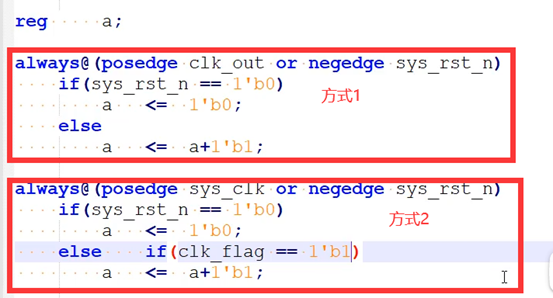二、实现 1.方式一分频器波形图 由于奇数分频器的特殊性，没有办法采取偶数分频器的方法，所以采取使其产生两个clk1与clk2，然后让其两个相与得到最终的五分频clk_out，波形图如下图所示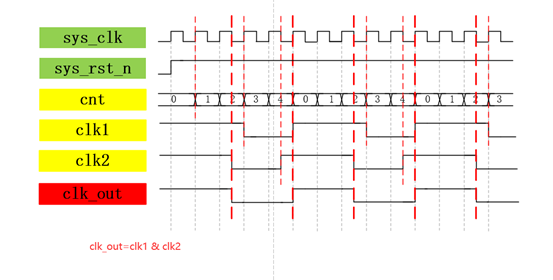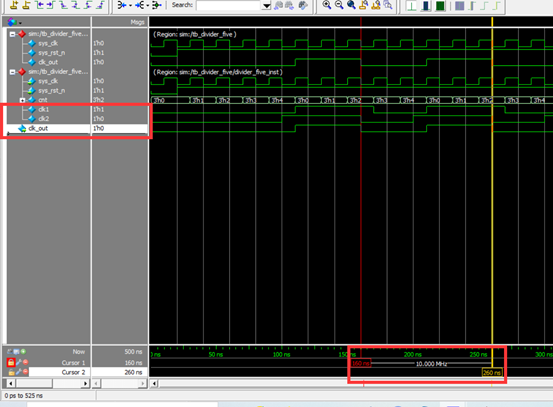2.程序 module divider_five ( input wire sys_clk , input wire sys_rst_n ,
output wire clk_out
);
reg [2:0] cnt; reg clk1; reg clk2;
always@(posedge sys_clk or negedge sys_rst_n) if(sys_rst_n == 1’b0) cnt <= 3’d0; else if (cnt == 3’d4) cnt <= 3’d0; else cnt <= cnt + 3’d1;
always@(posedge sys_clk or negedge sys_rst_n) if(sys_rst_n == 1’b0) clk1 <= 1’b0; else if (cnt == 3’d2) clk1 <= 1’b0; else if (cnt == 3’d4) clk1 <= 1’b1; else clk1 <= clk1;
always@(negedge sys_clk or negedge sys_rst_n) if(sys_rst_n == 1’b0) clk2 <= 1’b0; else if (cnt == 3’d2) clk2 <= 1’b0; else if (cnt == 3’d4) clk2 <= 1’b1; else clk2 <= clk2;
assign clk_out = (clk1 & clk2);
endmodule
仿真程序与六倍频仿真程序相似 timescale 1ns/1ns module tb_divider_five();
reg sys_clk; reg sys_rst_n;
wire clk_out;
initial begin sys_clk = 1’b0; sys_rst_n <= 1’b0; #20 sys_rst_n <= 1’b1; end
always #10 sys_clk = ~sys_clk;
initial begin \$timeformat(-9,0,“ns”,6);

m

o

n

i

t

o

r

(

"

@

t

i

m

e

monitor("@time %t:clk_out=%b",

time,clk_out); end
divider_five divider_five_inst ( .sys_clk (sys_clk) , .sys_rst_n(sys_rst_n) ,
.clk_out (clk_out)
); endmodule
2.方式二降频波形图 与六倍频相似，只是相差了一个相位，可对比得到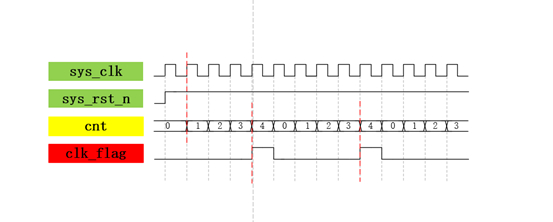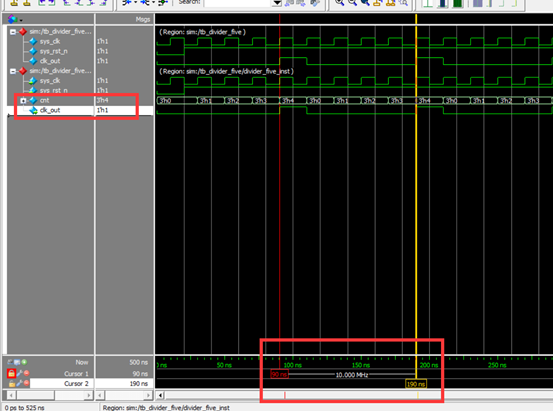3.程序 module divider_five ( input wire sys_clk, input wire sys_rst_n,
output reg clk_out
); reg [2:0] cnt;
always@(posedge sys_clk or negedge sys_rst_n) if(sys_rst_n == 1’b0) clk_out <= 1’b0; else if (cnt == 3’d3) clk_out <= 1’b1; else clk_out <= 1’b0;
always@(posedge sys_clk or negedge sys_rst_n) if(sys_rst_n == 1’b0) cnt <= 3’b0; else if(cnt == 3’d4) cnt <= 3’d0; else cnt <= cnt + 1’b1; endmodule
仿真程序与方式一没啥区别
展开全文编程语言 verilog
• VHDL Quartus 五分频器源代码 --半周期是原来半周期信号的N倍 --一个上升沿是2分频 --两个上升沿就是4分频 --三个上升沿就是6分频 LIBRARY IEEE; --调用库 USE IEEE.STD_LOGIC_1164.ALL;--调用包集合 USE IEEE.STD_...
• 可编程硬件描述语言VHDL Quartus 五分频器源代码.rar
• ## 分频器的Verilog实现

千次阅读 2020-07-05 11:20:46
分频器的Verilog实现 写在前面的话：找工作过程中经常遇到的分频类型，在此简单整理，代码亲自验证过，如有问题，烦请告知。 1.偶分频 偶分频比较简单，假设为N分频，只需计数到N/2－1，然后时钟翻转、计数清零，...
分频器的Verilog实现 写在前面的话：找工作过程中经常遇到的分频类型，在此简单整理，代码亲自验证过，如有问题，烦请告知。 1.偶分频 偶分频比较简单，对于N（N为偶数）分频，只需计数到N/2－1，然后时钟翻转、计数清零，如此循环就可以得到N（偶）分频。 （二分频） module div_2 (q,clk,rst_n); input rst_n; input clk; output reg q;
always @ (posedge clk or negedge rst_n)begin
if(rst_n==1'b0)
q<=1'b0;
else
q<=~q;
end

endmodule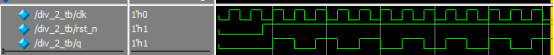二分频仿真结果图 （六分频） module div_6(clk_out,clk,rst_n);
 output clk_out;
input clk;
input rst_n;

reg [1:0] cnt;
reg clk_out;

parameter N=6;

always @ (posedge clk or negedge rst_n)begin
if(rst_n==1'b0)
begin
cnt <= 0;
clk_out<= 0;
end
else  if(cnt==N/2-1)
begin
clk_out<=~clk_out;
cnt<=0;
end
else
cnt <= cnt + 1'b1;
end

endmodule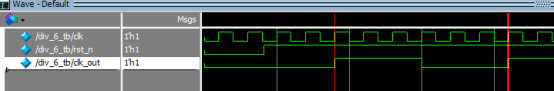六分频仿真结果图 2.奇分频（占空比50%） 实现奇数（N）分频，分别用上升沿计数到（N-1)/2，再计数到N-1；用下降沿计数到（N-1)/2，再计数到N-1，得到两个波形，然后把它们相或即可得到N分频。 （3分频） module div_3 (q,clk,rst_n); output q; input rst_n; input clk;
reg q1,q2;
reg [1:0] count1,count2;

always@(posedge clk or negedge rst_n)begin
if(rst_n==1'b0)
begin
q1<=1'b0;
count1<=2'b00;
end
else if(count1==0)
begin
q1<=~q1;
count1<=count1+1'b1;
end
else if(count1==1)
begin
q1=~q1;
count1<=count1+1'b1;
end
else
count1<=2'b00;
end

always@(negedge clk or negedge rst_n)begin
if(rst_n==1'b0)
begin
q2<=1'b0;
count2<=2'b00;
end
else if(count2==0)
begin
q2<=~q2;
count2<=count2+1'b1;
end
else if(count2==1'b1)
begin
q2=~q2;
count2<=count2+1'b1;
end
else
count2<=2'b00;
end
assign q=q1|q2;

endmodule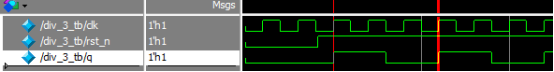3分频仿真图 （5分频）//5分频原理与3分频一样，只不过代码设计上简化了一点，不影响结果。 module test( input clk, input rst_n, output q );
reg [2:0] cnt;
reg q1, q2;

always@(posedge clk or negedge rst_n) begin
if(~rst_n) begin
cnt <= 0;
end
else if(cnt <3'd4) begin
cnt <= cnt + 1'b1;
end
else
cnt <= 0;

end

always@(posedge clk or negedge rst_n) begin
if(~rst_n) begin
q1 <= 1'b1;
end
else if(cnt == 3'd1) begin
q1 <= ~q1;
end
else if(cnt == 3'd4) begin
q1 <= ~q1;
end
else	q1 <= q1;
end

always@(negedge clk or negedge rst_n) begin
if(~rst_n) begin
q2 <= 1'b1;
end
else q2 <= q1;
end

assign q = q1 | q2;

endmodule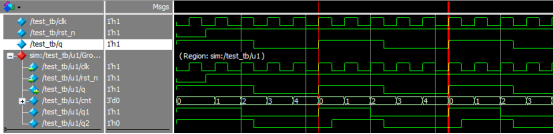5分频仿真结果图 3.占空比不为50% 在此拿三分频举例，分别给出1/3、2/3占空比例子。对于任意占空比首先要知道占空比是高电平与周期之比，然后通过计数产生高电平个数、取反，再计数到N-1取反，计数器置零。 （1）占空比2/3 module div3(clk,rst_n,q);
input			clk;
input			rst_n;
output	reg	q;

reg [1:0] cnt;

always@(posedge clk or negedge rst_n)begin
if(rst_n==1'b0)
begin
cnt<=2'd0;
q<=0;
end
else if(cnt==2'd0)
begin
q<=~q;
cnt<=cnt+1'b1;
end
else if(cnt==2'd2)
begin
q<=~q;
cnt<=2'd0;
end
else begin
q<=q;
cnt<=cnt+1'b1;
end
end

endmodule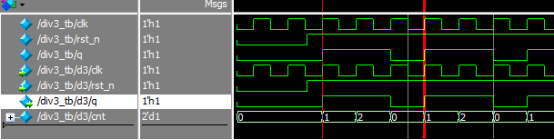占空比2/3仿真图 （2）占空比1/3 module div3(clk,rst_n,q);
input			clk;
input			rst_n;
output	reg	q;

reg [1:0] cnt;

always@(posedge clk or negedge rst_n)begin
if(rst_n==1'b0)
begin
cnt<=2'd0;
q<=0;
end
else if(cnt==2'd1)//代码仅此处计数值不同
begin
q<=~q;
cnt<=cnt+1'b1;
end
else if(cnt==2'd2)
begin
q<=~q;
cnt<=2'd0;
end
else begin
q<=q;
cnt<=cnt+1'b1;
end
end

endmodule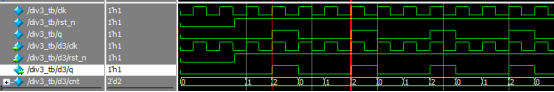占空比1/3 通过以上1/3、2/3可以知道对于其余不同占空比N分频应该可以有所理解。
展开全文verilog
• 基于VHDL语言分频器电路程序设计（汇总） 分频器简介： 分频器是数字电路中最常用的电路之一，在 FPGA 的设计中也是使用效率非常高的基本设计。基于 FPGA 实现的分频电路一般有两种方法：一是使用FPGA 芯片内部提供...
基于VHDL语言分频器电路程序设计（汇总）
分频器简介：
分频器是数字电路中最常用的电路之一，在 FPGA 的设计中也是使用效率非常高的基本设计。基于 FPGA 实现的分频电路一般有两种方法：一是使用FPGA 芯片内部提供的锁相环电路，如 ALTERA 提供的 PLL（Phase Locked
Loop），Xilinx 提供的 DLL（Delay Locked Loop）；二是使用硬件描述语言，如VHDL、Verilog HDL 等。使用锁相环电路有许多优点，如可以实现倍频；相位偏移；占空比可调等。但 FPGA 提供的锁相环个数极为有限，不能满足使用要求。因此使用硬件描述语言实现分频电路经常使用在数字电路设计中，消耗不多的逻辑单元就可以实现对时钟的操作，具有成本低、可编程等优点。
计数器
计数器是实现分频电路的基础，计数器有普通计数器和约翰逊计数器两种。这两种计数器均可应用在分频电路中。
普通计数器： 最普通的计数器是加法（或减法）计数器。约翰逊计数器： 约翰逊计数器是一种移位计数器，采用的是把输出的最高位取非，然后反馈送到最低位触发器的输入端。约翰逊计数器在每个时钟下只有一个输出发生变化。
分频器
如前所述，分频器的基础是计数器，设计分频器的关键在于输出电平翻转的时机。下面使用加法计数器分别描述各种分频器的实现。
偶数分频器：偶数分频最易于实现，欲实现占空比为 50%的偶数 N 分频，一般来说有两种方案：一是当计数器计数到N/2-1 时，将输出电平进行一次翻转，同时给计数器一个复位信号，如此循环下去；二是当计数器输出为 0 到 N/2-1 时，时钟输出为 0 或 1，计数器输出为 N/2 到 N-1 时，时钟输出为 1 或 0，当计数器计数到N-1 时，复位计数器，如此循环下去。需要说明的是，第一种方案仅仅能实现占空比为 50%的分频器，第二种方案可以有限度的调整占空比，参考非 50%占空比的奇数分频实现。奇数分频器：实现非50%占空比的奇数分频，如实现占空比为 20%（1/5）、40%（2/5）、60%（3/5）、80%（4/5）的 5 分频器，可以采用似偶数分频的第二种方案；但如果实现占空比为 50%的奇数分频，就不能使用偶数分频中所采用的方案了。半整数分频器：仅仅采用数字分频，不可能获得占空比为 50%的 N+0.5 分频，我们只可以设计出占空比为(M+0.5)/(N+0.5)或者 M/(N+0.5)的分频器，M 小于 N。这种半整数分频方法是对输入时钟进行操作，让计数器计数到某一个数值时，将输入时钟电平进行一次反转，这样，该计数值只保持了半个时钟周期，因此实现半整数分频。小数分频器：小数分频是通过可变分频和多次平均的方法实现的。例如要实现 4.7 分频，只要在 10 次分频中，做 7 次 5 分频，3 次 4 分频就可以得到。再如要实现 5.67 分频，只要在 100 次分频中，做 67 次6 分频，33 次 5 分频即可。考虑到小数分频器要进行多次两种频率的分频，必须设法将两种分频均匀。分数分频器：将小数分频的方法进行扩展，可以得到形如M (L/N )的分数分频的方法，例如， 2(7/13)等于分母的，进行分频，只要在 13 次分频中，进行 7 次 3 分频，6 次 2 分频就可以得到。同样，为了将两种分频均匀，将分子部分累加，小于分母的，进行M分频，大于(M+1)分频。积分分频器:积分分频器用于实现形如

2

m

−

1

/

N

2^{m-1}/N

的分频，例如 8/3 分频。我们当然可以使用上面提到的分数分频的方法，但对于这种形式的分频，使用积分分频的方法综合往往占用更少的 FPGA 资源。积分分频法基于下述原理：一个 m 位的二进制数字每次累加 N，假定累加x 次累加值最低m 位回到 0，同时越过

2

m

y

2^my

次，那么，当前累加的数字应该是Nx=

2

m

y

2^my

；每越过

2

m

2^m

一次，最高位变化 2 次，所以，累加 x 次，最高位变化 2y次，得到

x

/

2

y

=

2

m

−

1

/

N

x/2y=2^{m-1}/N

分频的分频器例如，取 m 为 4，N 为 3，当累加 16 次时，累加值为 48，最低 m 位变回到 0，同时越过 16 三次，最高位变化 6 次，由此得到 16/6=8/3 分频的分频器。
注意： 以上分频器程序设计的案例将会在下边进行一一分析。
软件说明： ModelSimSetup-13.1.0.162，QuartusSetup-13.1.0.162。
建立工程：
第一步：打开Quartus软件。
第二步：点击New Project Wizard -> next.
第三步：选择工程文件的存放位置，输入工程名 -> next -> next。
第四步：在family栏选择芯片型号-Cyclone IV E，在Name栏选择EP4CE115F29C7，选择完之后点击next。(如果不进行硬件调试时，此处默认即可)
第五步：检查工程有没有建错，点击完成。如下图：程序设计：
普通计数器：
--文件名：ADDER8B.vhd 应与工程名保持一致：
--Description: 带复位功能的加法计数器
library ieee;
use ieee.std_logic_1164.all;
use ieee.std_logic_arith.all;
use ieee.std_logic_unsigned.all;
entity ripple is
generic (width: integer := 4);
port(clk, rst: in std_logic;
cnt: out std_logic_vector(width - 1 downto 0));
end ripple;
architecture a of ripple is
signal cntQ: std_logic_vector(width - 1 downto 0);
begin
process(clk, rst)
begin
if (rst = '1') then
cntQ <= (others => '0');
elsif (clk'event and clk = '1') then
cntQ <= cntQ + 1;
end if;
end process;
cnt <= cntQ;
end a;

在同一时刻，加法计数器的输出可能有多位发生变化，因此，当使用组合逻辑对输出进行译码时，会导致尖峰脉冲信号。使用约翰逊计数器可以避免这个问题。
文件仿真(这里采用modelsim仿真波形)：
选择File-> New -> Verification/Debugging Files ->University Program VWF。2.打开测试文件。(右键点击添加端口，对输入信号初始化，赋值。)3.仿真结果：逻辑电路图：约翰逊计数器：
--file Name: johnson.vhd
--Description: 带复位功能的约翰逊计数器
library ieee;
use ieee.std_logic_1164.all;
use ieee.std_logic_arith.all;
use ieee.std_logic_unsigned.all;
entity johnson is
generic (width: integer := 4);
port (clk, rst: in std_logic;
cnt: out std_logic_vector(width - 1 downto 0));
end johnson;
architecture a of johnson is
signal cntQ: std_logic_vector(width - 1 downto 0);
begin
process(clk, rst)
begin
if(rst = '1') then
cntQ <= (others => '0');
elsif (rising_edge(clk)) then
cntQ(width - 1 downto 1) <= cntQ(width - 2 downto 0);
cntQ(0) <= not cntQ(width - 1);
end if;
end process;
cnt <= cntQ;
end a;


逻辑电路图：仿真结果：显然，约翰逊计数器没有有效利用寄存器的所有状态，假设最初值或复位状态为0000，则依次为 0000、0001、0011、0111、1111、1110、1100、1000、0000 如 循环。再者，如果由于干扰噪声引入一个无效状态，如 0010，则无法恢复到有效到循环中去，需要我们加入错误恢复处理.
偶数分频器:(6 分频)
architecture a 使用的是第一种方案，architecture b 使用的是第二种方案。更改 configuration 可查看不同方案的综合结果。
--filename clk_div6.vhd
--description: 占空比为 50%的 6 分频
library ieee;
use ieee.std_logic_1164.all;
use ieee.std_logic_arith.all;
use ieee.std_logic_unsigned.all;
entity clk_div6 is
port(clk_in: in std_logic; clk_out: out std_logic);
end clk_div6;
--使用第一种方案
architecture a of clk_div6 is
signal clk_outQ: std_logic := '0';--赋初始值仅供仿真使用
signal countQ: std_logic_vector(2 downto 0) := "000";
begin
process(clk_in)
begin
if(clk_in'event and clk_in = '1') then
if(countQ /= 2) then
CountQ <= CountQ + 1;
else
clk_outQ <= not clk_outQ;
CountQ <= (others =>'0');
end if;
end if;
end process;
clk_out <= clk_outQ;
end a;
--使用第二种方案
architecture b of clk_div6 is
signal countQ: std_logic_vector(2 downto 0);
begin
process(clk_in)
begin
if(clk_in'event and clk_in = '1') then
if(countQ < 5) then
countQ <= countQ + 1;
else
CountQ <= (others =>'0');
end if;
end if;
end process;
process(countQ)
begin
if(countQ < 3) then
clk_out <= '0';
else
clk_out <= '1';
end if;
end process;
end b;

configuration cfg of clk_div6 is
for a
end for;
end cfg;

逻辑电路图：
architecture a:architecture b:仿真结果：
architecture a、b:奇数分频器：
非 50%占空比：
下面就以实现占空比为40%的 5 分频分频器为例，说明非 50%占空比的奇数分频器的实现。该分频器的实现对于我们实现 50%占空比的分频器有一定的借鉴意义。
--filename clk_div5.vhd
--description: 占空比为 40%的 5 分频
library ieee;
use ieee.std_logic_1164.all;
use ieee.std_logic_arith.all;
use ieee.std_logic_unsigned.all;
entity clk_div5 is
port(clk_in: in std_logic; clk_out: out std_logic);
end clk_div5;
architecture a of clk_div5 is
signal countQ: std_logic_vector(2 downto 0);
begin
process(clk_in)
begin
if(clk_in'event and clk_in = '1') then
if(countQ < 4) then
countQ <= countQ + 1;
else
CountQ <= (others =>'0');
end if;
end if;
end process;
process(countQ)
begin
if(countQ < 3) then
clk_out <= '0';
else
clk_out <= '1';
end if;
end process;
end a;

逻辑电路图：仿真结果：50%占空比的奇数分频:
通过待分频时钟下降沿触发计数，产生一个占空比为40%（2/5）的 5 分频器。将产生的时钟与上升沿触发产生的时钟相或，即可得到一个占空比 50%的 5 分频器。
推广为一般方法：欲实现占空比为 50%的 2N+1 分频器，则需要对待分频时钟上升沿和下降沿分别进行N/(2N+1)分频，然后将两个分频所得的时钟信号相或得到占空比为 50%的 2N+1 分频器。
下面的代码就是利用上述思想获得占空比为 50%的 7 分频器。需要我们分别对上升沿和下降沿进行 3/7 分频，再将分频获得的信号相或。
--filename clk_div7.vhd
--description: 占空比为 50%的 7 分频
library ieee;
use ieee.std_logic_1164.all;
use ieee.std_logic_arith.all;
use ieee.std_logic_unsigned.all;
entity clk_div7 is
port(clk_in: in std_logic; clk_out: out std_logic);
end clk_div7;

architecture a of clk_div7 is
signal cnt1, cnt2: integer range 0 to 6;
signal clk1,clk2: std_logic;
begin
process(clk_in)--上升沿
begin
if(rising_edge(clk_in)) then
if(cnt1 < 6)then
cnt1 <= cnt1 + 1;
else
cnt1 <= 0;
end if;
if(cnt1 < 3) then
clk1 <= '1';
else
clk1 <= '0';
end if;
end if;
end process;
process(clk_in)--下降沿
begin
if(falling_edge(clk_in)) then
if(cnt2 < 6) then
cnt2 <= cnt2 + 1;
else
cnt2 <= 0;
end if;
if(cnt2 < 3) then
clk2 <= '1';
else
clk2 <= '0';
end if;
end if;
end process;
clk_out <= clk1 or clk2;
end a;

逻辑电路图：仿真结果：半整数分频器:
如上所述，占空比为 50%的奇数分频可以帮助我们实现半整数分频，将占空比为50%的奇数分频与待分频时钟异或得到计数脉冲，下面的代码就是依靠占空比为 50%的 5 分频实现 2.5 分频器的。
--filename clk_div2_5.vhd
--description: 占空比为 1/1.5，即 60%。的 2.5分频
library ieee;
use ieee.std_logic_1164.all;
use ieee.std_logic_arith.all;
use ieee.std_logic_unsigned.all;

entity clk_div2_5 is
port(clk_in: in std_logic; clk_out: out std_logic);
end clk_div2_5;

architecture a of clk_div2_5 is
signal cnt1, cnt2: integer range 0 to 4;
signal clk1, clk2: std_logic;
signal Pclk, Lclk: std_logic;
signal cnt3:integer range 0 to 2;
begin
process(clk_in)
begin
if(rising_edge(clk_in)) then
if(cnt1 < 4) then
cnt1 <= cnt1 + 1;
else
cnt1 <= 0;
end if;
end if;
end process;
process(clk_in)
begin
if(falling_edge(clk_in)) then
if(cnt2 <4)  then
cnt2 <= cnt2 + 1;
else
cnt2 <= 0;
end if;
end if;
end process;
process(cnt1)
begin
if (cnt1 <3) then
clk1 <= '0';
else
clk1 <= '1';
end if;
end process;
process(cnt2)
begin
if (cnt2 < 3) then
clk2 <= '0';
else
clk2 <= '1';
end if;
end process;

process(Lclk)
begin
if(rising_edge(Lclk)) then
if(cnt3 < 2) then
cnt3 <= cnt3 + 1;
else
cnt3 <= 0;
end if;
end if;
end process;
process(cnt3)
begin
if(cnt3 < 2) then
clk_out <= '0';
else
clk_out <='1';
end if;
end process;
Pclk <= clk1 or clk2;
Lclk <= clk_in xor Pclk;--对输入时钟进行处理
end a;

仿真结果：小数分频器:
表 1以 2.7 分频为例，小数部分进行累加，如果大于等于10，则进行 3 分频，如果小于 10，进行
2 分频。
表一：小数分频系数序列
序号0123456789累加值71411815129161310分频 系数2332332333
下加器面的代码就是基于上述原理实现 2.7 分频。architecture b 是使用累加器计算分频系数选则时机， chitectur a 是直接使用已计算好的结果。
--file name: clk_div2_7.vhd
--description: 2.7 分频 ,占空比应为 10/27。
library ieee;
use ieee.std_logic_1164.all;
use ieee.std_logic_arith.all;
use ieee.std_logic_unsigned.all;
entity clk_div2_7 is
port(clk_in: in std_logic; clk_out: out std_logic);
end clk_div2_7;
architecture b of clk_div2_7 is
signal clkoutQ: std_logic;
signal ctrl: std_logic;
signal cnt1: integer range 0  to 1;
signal cnt2: integer range 0  to 2;
begin
clk_out <= clkoutQ;
process(clkoutQ)
variable tmp: integer range 0 to 20;
begin
if(rising_edge(clkoutQ)) then
tmp := tmp + 7;
if(tmp < 10) then
ctrl <= '1';
else
ctrl <= '0';
tmp := tmp - 10;
end if;
end if;
end process;

process(clk_in)
begin
if(clk_in'event and clk_in = '1') then
if(ctrl = '1') then
if(cnt1 < 1) then
cnt1 <= cnt1 + 1;
else
cnt1 <= 0;
end if;
if(cnt1 < 1) then
clkoutQ <= '1';
else
clkoutQ <= '0';
end if;
else
if(cnt2 < 2) then
cnt2 <= cnt2 + 1;
else
cnt2 <= 0;
end if;
if(cnt2 < 1) then
clkoutQ <= '1';
else
clkoutQ <= '0';
end if;
end if;
end if;
end process;
end b;
architecture a of clk_div2_7 is
signal cnt: integer range 0 to 9;
signal clkoutQ: std_logic;
signal cnt1: integer range 0 to 1;
signal cnt2: integer range 0 to 2;
begin
clk_out <= clkoutQ;
process(clkOutQ)
begin
if(clkoutQ'event and clkoutQ = '1') then
if (cnt < 9) then
cnt <= cnt + 1;
else
cnt <= 0;
end if;
end if;
end process;
process(clk_in)
begin
if(clk_in'event and clk_in = '1') then
case cnt is
when 0|3|6 =>
if(cnt1 < 1) then
cnt1 <= cnt1 + 1;
else
cnt1 <= 0;
end if;
if(cnt1 < 1) then
clkoutQ <= '1';
else
clkoutQ <='0';
end if;
when others =>
if(cnt2 < 2) then
cnt2 <= cnt2 + 1;
else
cnt2 <= 0;
end if;
if(cnt2 < 1) then
clkoutQ <= '1';
else
clkoutQ <= '0';
end if;
end case;
end if;
end process;
end a;

configuration cfg of clk_div2_7 is
for a
end for;
end cfg;

仿真结果：分数分频器:
表 2显示了 2(7/13)的分频次序。仿照小数分频器代码，给出 2(7/13) 分频的代码如下：
表 2 分数分频系数序列
序号0123456789101112累加值71481591610171118121913分频 系数2323232323233
--file name: clk_div2_7_13.vhd
--description: 33/13分频
library ieee;
use ieee.std_logic_1164.all;
use ieee.std_logic_arith.all;
use ieee.std_logic_unsigned.all;
entity clk_div2_7 is
port(clk_in: in std_logic; clk_out: out std_logic);
end clk_div2_7;
architecture b of clk_div2_7 is
signal clkoutQ: std_logic;
signal ctrl: std_logic;
signal cnt1: integer range 0  to 1;
signal cnt2: integer range 0  to 2;
begin
clk_out <= clkoutQ;
process(clkoutQ)
variable tmp: integer range 0 to 26;
begin
if(rising_edge(clkoutQ)) then
tmp := tmp + 7;
if(tmp < 10) then
ctrl <= '1';
else
ctrl <= '0';
tmp := tmp - 13;
end if;
end if;
end process;

process(clk_in)
begin
if(clk_in'event and clk_in = '1') then
if(ctrl = '1') then
if(cnt1 < 1) then
cnt1 <= cnt1 + 1;
else
cnt1 <= 0;
end if;
if(cnt1 < 1) then
clkoutQ <= '1';
else
clkoutQ <= '0';
end if;
else
if(cnt2 < 2) then
cnt2 <= cnt2 + 1;
else
cnt2 <= 0;
end if;
if(cnt2 < 1) then
clkoutQ <= '1';
else
clkoutQ <= '0';
end if;
end if;
end if;
end process;
end b;
architecture a of clk_div2_7 is
signal cnt: integer range 0 to 12;
signal clkoutQ: std_logic;
signal cnt1: integer range 0 to 1;
signal cnt2: integer range 0 to 2;
begin
clk_out <= clkoutQ;
process(clkOutQ)
begin
if(clkoutQ'event and clkoutQ = '1') then
if (cnt < 9) then
cnt <= cnt + 1;
else
cnt <= 0;
end if;
end if;
end process;
process(clk_in)
begin
if(clk_in'event and clk_in = '1') then
case cnt is
when 0|2|4|6|8|10 =>
if(cnt1 < 1) then
cnt1 <= cnt1 + 1;
else
cnt1 <= 0;
end if;
if(cnt1 < 1) then
clkoutQ <= '1';
else
clkoutQ <='0';
end if;
when others =>
if(cnt2 < 2) then
cnt2 <= cnt2 + 1;
else
cnt2 <= 0;
end if;
if(cnt2 < 1) then
clkoutQ <= '1';
else
clkoutQ <= '0';
end if;
end case;
end if;
end process;
end a;

configuration cfg of clk_div2_7 is
for b
end for;
end cfg;


仿真结果：积分分频器:
例如，取 m 为 4，N 为 3，当累加 16 次时，累加值为 48，最低 m 位变回到 0，同时越过 16 三次，最高位变化 6 次，由此得到 16/6=8/3 分频的分频器。
--file name: clk_div8.vhd
--description: 使用积分分频实现 8/3 分频
library ieee;
use ieee.std_logic_1164.all;
use ieee.std_logic_unsigned.all;
entity clk_div_8f3 is
port(clk_in: in std_logic; clk_out: out std_logic);
end clk_div_8f3;
architecture a of clk_div_8f3 is
signal cnt: std_logic_vector(3 downto 0) := (others => '0');
signal dly: std_logic;
begin
process(clk_in)
begin
if(clk_in'event and clk_in = '1') then
dly <= cnt(3);
cnt <= cnt + 3;
end if;
end process;
clk_out <= dly xor cnt(3);
end a;

仿真结果：展开全文fpga 小数分频 奇偶分频
• ## D触发器实现分频器

千次阅读 2021-04-27 11:54:10
D触发器实现分频器 对于分频器的实现，我们最常用的方法就是通过计数器来实现，具体的实现可以看前面的文章，但是通过D触发器实现却很少遇到，我将通过这篇文章讲解D触发器实现分频器，这里以9分频为例。 我们要实现...
D触发器实现分频器
对于分频器的实现，我们最常用的方法就是通过计数器来实现，具体的实现可以看前面的文章，但是通过D触发器实现却很少遇到，我将通过这篇文章讲解D触发器实现分频器，这里以9分频为例。
我们要实现的是通过D触发器和组合逻辑实现占空比为50%的9分频电路，首先我们通过D触发器实现序列发生器，输出000001111循环序列，然后用下降沿的D触发器打一拍，将两个信号相或后输出即可。
通过上面的思路，我们首先画出对应的波形图，其中clk1为序列发生器产生的序列脉冲，clk2是clk1在系统时钟clk的下降沿打一拍得到的信号，div_clk为我们得到的9分频信号。现在的关键就在于如何产生000001111循环序列，因为该循环序列有9个状态，所以至少需要4个触发器，所以我们首先使用4个触发器列出对应的状态：
Q3Q2Q1Q0D00001000110011101111111101110011000100000000 ？？？0
此时我们看到使用四个触发器时，我们在产生循环序列时，当产生第5个0时，发现触发器的取值和产生第一个1时的取值相同，所以，不能用四个触发器来产生该循环序列，为了满足条件，我们使用五个触发器，如下表：
Q4Q3Q2Q1Q0D000001000011000111001111011110111100111000110000100000000001
从上面可以看到，五个触发器可以满足条件，在确定了触发器的个数之后，我们就要通过上表来画出对应发的卡诺图，然后进行化简，得到状态方程，然后根据状态方程画出电路。对应的卡诺图如下：在化简的时候，没有数据的地方，我们既可以看做1，也可以看做0，最后的化简结果为D=Q4‘Q3’=(Q4+Q3)’，根据状态方程就可以设计电路图了，电路图如下：得到电路图后，下面给出完整的代码：
// -----------------------------------------------------------------------------
// -----------------------------------------------------------------------------
// Author : dongtaolv
// Email  : tdlv@stu.xidian.edu.cn
// File   : div9_clk.v
// Create : 2021-04-27 11:46:24
// Revise : 2021-04-27 11:46:24
// Editor : sublime text3, tab size (4)
// -----------------------------------------------------------------------------

module div9_clk(
input	wire	clk,
input	wire	rst,
output	wire	div_clk
);

reg 	Q0,Q1,Q2,Q3,Q4;
wire	D0;
reg		out_reg;

always @(posedge clk)begin
if(rst)begin
Q0 <= 1'b0;
Q1 <= 1'b0;
Q2 <= 1'b0;
Q3 <= 1'b0;
Q4 <= 1'b0;
end
else begin
Q0 <= D0;
Q1 <= Q0;
Q2 <= Q1;
Q3 <= Q2;
Q4 <= Q3;
end
end

always @(negedge clk)begin
if(rst)begin
out_reg <= 1'b0;
end
else begin
out_reg <= Q4;
end
end

assign D0 = ~(Q3 | Q4);

assign div_clk = out_reg | Q4;
endmodule

tb文件如下：
// -----------------------------------------------------------------------------
// -----------------------------------------------------------------------------
// Author : dongtaolv
// Email  : tdlv@stu.xidian.edu.cn
// File   : tb_div9_clk.v
// Create : 2021-04-27 11:47:34
// Revise : 2021-04-27 11:47:34
// Editor : sublime text3, tab size (4)
// -----------------------------------------------------------------------------
timescale 1ns/1ps

module tb_div9_clk;

reg		clk;
reg		rst;
wire	div_clk;

initial begin
clk = 0;
rst = 1;
#100
rst = 0;
end

always #10 clk = ~clk;

div9_clk inst_div9_clk (
.clk(clk),
.rst(rst),
.div_clk(div_clk)
);

endmodule

仿真图如下：其中，clk为系统时钟，div_clk为9分频的时钟。
展开全文• FPGA基础入门篇(七) 多路分频器的实现——任意整数分频器（二） 本次介绍分频的主要设计方法，如得到任意整数的分频器该怎么设计。前面介绍了2、3、4、8分频的设计方法。其实本质是利用计数器对时钟上升沿进行计数...
• 分频器是FPGA设计中使用频率非常高的基本设计之一，尽管在目前大部分设计中，广泛使用芯片厂家集成的锁相环资源，如赛灵思（Xilinx）的DLL.来进行时钟的分频，倍频以及相移。 但是对于时钟要求不高的基本设计，通过...
• ## 双模预分频器

千次阅读 热门讨论 2019-05-21 21:09:22
参考来源：A 1.75-GHz 3-V Dual-...双模预分频器的两种：第一种是传统的数字逻辑。 这个电路仔细仿了一下，还是很精巧的。 首先是怎么实现5分频。上面的Divide-by-4/5，当Ctrl信号是低的时候，最后一级始终...
• 分频器，顾名思义，就是将一个波形，分成具有若干占空比的波。占空比是指在一个脉冲循环内，通电时间相对于总时间所占的比例。占空比(Duty Ratio)在电信领域中有如下含义:例如:脉冲宽度1μs，信号周期4μs的脉冲序列...verilog FPGA
• 分频器，就是将特定频率的信号，通过计数分频的方式，得到自己需要的频率的信号。比如假如你的系统时钟是50Mhz,而你需要得到一个25Mhz的信号，则可以设计一个二分频模块对系统时钟进行分频获得。设计方法很简单，...fpga vhdl
• 分频器是指使输出信号频率为输入信号频率整数分之一的电子... 早期的分频器多为正弦分频器，随着数字集成电路的发展，脉冲分频器（又称数字分频器）逐渐取代了正弦分频器。 下面以Verilog HDL 语言为基础介绍占空比...
• 前言 将笔者之前在学习FPGA上遇到的一些有...分频器的设计通常分为以下三类：奇数分频器、偶数分频器及小数分频器。本文接下来将分别对奇数分频器及偶数分频器的设计仿真进行说明。 2.偶数分频器 2.1、说明 偶数分.
• 50MHZ 分频至1MHZ,1KHz,1Hz 分频器
• 这是一个基于QuartusII 9.0的一个分频器的verilog代码 非常适合初学者
• 从零开始的FPGA学习6偶数分频器奇数分频器完整分频器代码仿真波形图结束语 偶数分频器 假设为 N 分频，由待分频时钟触发计数器计数，当计数器从 0 计数到 N/2-1 时，输出时钟进行翻转，并给计数器一个复位信号，使得...
• 时钟整数分频分为奇数和偶数分频，偶数分频较为简单，假如需要进行偶数为N倍分频，则只需对原输入时钟进行从零开始计数count，当计数值count计数到N/2-1，只需将输出时钟反向即可，RTL代码和测试波形如下： ...
• ## 占空比为50%的奇数分频器的设计

千次阅读 多人点赞 2013-09-05 19:39:00
占空比为50%的奇数分频器设计思路  1. 首先进行上升沿触发的N模计数，计数器从零开始，到 (N-1)/2 处时钟翻转，然后经过 (N+1)/2再次翻转  得到一个占空比非50%的输出时钟out1.  2. 同时进行下降沿触发的N模计数...FPGA verilog
• N分频器，包括奇分频和偶分频，50%duty。 利用上升沿和下降沿分别生成的分频时钟clk_p,clk_n，占空比为（divisor&gt;&gt;1）/divisor，相或操作后，可以得到占空比50%的奇分频。 利用计数器在(cnt == 0) ...奇分频 偶分频
• 法一：可以通过待分频时钟下降沿触发计数，和上升沿同样的方法计数进行三分频，然后下降沿产生的三分频时钟和上升沿产生的时钟进行相或运算，即可得到占空比为50%的三分频时钟。 法二：对进行奇数倍n分频时钟，首先...verilog
• 在FPGA的学习过程中，最简单最基本的实验应该就是分频器了。由于FPGA的晶振频率都是固定值，只能产生固定频率的时序信号，但是实际工程中我们需要各种各样不同频率的信号，这时候就需要对晶振产生的频率进行分频。.....
• FPGA基础入门篇(七) 多路分频器的实现（一） FPGA基础入门篇(七) 多路分频器的实现（一） 本次实验实现多路分频器的设计，包括2分频、3分频、4分频、8分频，及2Hz的信号的实现。时钟采用100Mhz的系统系统时钟。 并...
• ... 1 偶数分频 （1）2的幂分频 ...i....i....图11 分频的RTL视图 ...图12 分频的仿真波形 ...N倍偶数分频器.(Verilog) http://blog.ednchina.com/2006tx_yafeng/146525/message.aspx 转载于:https...
• 因为这周有个作业要求用3个160实现一个类似2000分频占空比50的分频器，然后没用过verilog，就直接从网上抄了一个，但是并不好使，索性自己来 module LS160(clk,ep,et,ld,clr,dn,qn,cout); input clk,ep,et,ld,clr; ...
• 文章目录一、占空比不是50%（<<50%）二、占空比接近50%（>50%）三、占空比等于50%    奇数分频根据占空比的不同，写法也不尽相同。 一、占空比不是50%（<<50%） 1 module test#(parameter N=3)( ...
• 5.设计一个11分频的分频器，要求输出占空比为50%，不能使用PLL 法一 author : Mr.Mao e-mail : 2458682080@qq.com module div11x ( input clk, input reset_n, output q ); reg [3:0] cnt; reg x_p,x_n; ...verilog
• 2017年综合测评仿真电路讲解： ...常用电路Multisim仿真——数字芯片74LS74构建分频器设计： https://blog.csdn.net/DengFengLai123/article/details/99238311 常用电路Multisim仿真——仪器仪表使用： ...数字电路 电子设计竞赛
• 我们实验课老师为了加强实验难度，把实验题改掉了，用74LS112(或者74LS74)设计一个十四分频器（原来是十六分频器）。我稍微思考了一下，其实挺简单的。 用JK触发器，D触发器，做2的幂数的分频器是很简单的，只要...设计
• 方法：先实现一个按时钟上升沿的五分频器，同样的分频方法，实现一个以下降沿触发的五分频器，然后把两个分频器的输出相或，就可以得到占空比为50%的五分频电路。 `timescale 1ns / 1ps /////////////////////////...output input comments module...